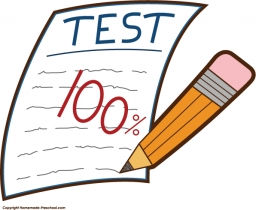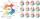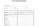# TV competition

In the competition, 10 contestants answer five questions, one question per round. Anyone who answers correctly will receive as many points as the number of competitors answered incorrectly in that round.

After the contest, one of the contestants said: We got 116 points in total, of which I was 30.

a. In how many rounds did all competitors respond correctly?
b. In how many rounds did at least 5 competitors respond correctly?
c. In how many rounds did 3 competitors respond correctly?

O1 =  0
O2 =  2
O3 =  2

### Step-by-step explanation:Did you find an error or inaccuracy? Feel free to write us. Thank you!Tips to related online calculators
Looking for help with calculating roots of a quadratic equation?
Do you have a linear equation or system of equations and looking for its solution? Or do you have a quadratic equation?
Do you solve Diofant problems and looking for a calculator of Diofant integer equations?

## Related math problems and questions:

• ContestantsIn the competition it was possible to get 0-5 points. In fact, 5 contestants of the 15 best contestants has earned 5 points or 4 points (which have won 10 contestants). The number of contestants who earned 3 points was the same as the number of contestant
• Math testObelix filled a mathematical test in which he answered 25 questions. For every correct answer, he received 5 points, for each bad answer he had 3 points deducted. Obelix gained 36% of all points in the test. How many questions did he solve correctly?
• Petra's testThe answer will be given 8 marks, and the wrong answer will be deducted 3 marks. The total number of marks obtained by the school is 36. If Petra answered six questions correctly,   how many questions were answered incorrectly by the student from the scho
• Intelligence testPaľo, Jano, Karol, and Rišo were doing an intelligence test. Palo correctly answered half of the questions plus 7 questions, Jano to a third plus 18 questions, Karol to a quarter plus 21 questions and Risho to a fifth plus 25 questions. After the test, Ka
• TestThe teacher prepared a test with ten questions. The student can choose one correct answer from the four (A, B, C, D). The student did not get a written exam at all. What is the probability that: a) He answers half correctly. b) He answers all correctly. c
• Six questions testThere are six questions in the test. There are 3 answers to each - only one is correct. In order for a student to take the exam, at least four questions must be answered correctly. Alan didn't learn at all, so he circled the answers only by guessing. What
• Divisible by 5How many three-digit odd numbers divisible by 5, which are in place ten's number 3?
• CompetitorsIn the first round of slalom fell 15% of all competitors and in the second round another ten racers. Together, 40% of all competitors fell. What was the total number of competitors?
• QuizTested student answered correctly on a 4/ 8 questions. Wrong answers was 16. How many questions answered?
• Unknown number 10Number first increased by 30%, then by 1/5. What percentage we've increased the original number?
• TestingStudents of high school have 10 points for each good solved task. The wrong answer is deducted by 5 points. After solving 20 tasks, student Michael had 80 points. How many tasks did he solve correctly and how many wrong?
• Quiz or testI have a quiz with 20 questions. Each question has 4 multiple choice answers, A, B, C, D. THERE IS NO WAY TO KNOW THE CORRECT ANSWER OF ANY GIVEN QUESTION, but the answers are static, in that if the "correct" answer to #1 = C, then it will always be equal
• StudentsStudents solve word problems. They received 3 points for each correctly solved task. Four points were deducted for a task that was not solved or incorrectly solved. Tomas solved a total of 15 tasks and scored 24 points. How many tasks did he solve correct
• Final examAt the final exam, the student answers from three areas, which are evaluated in a ratio of 1: 2: 2. What grade will John receive if he answered as follows: 3,1,2.Divide number 135 into two additions so that one adds 30 more than 2/5 of the other add. Write the bigger one.Three friends divided the profit 104,650 CZK, so that for every 4 CZK, which got the first friend equals 5 crowns for second and for every 9 CZK, which got the second equals 16 CZK for third. Question: Who got the most and how much.The test contains four questions, and there are five different answers to each of them, of which only one is correct, the others are incorrect. What is the probability that a student who does not know the answer to any question will guess the right answer